# Plane (geometry)

In Euclidean geometry, a plane is an abstract concept that models the common notion of a flat surface — without depressions, protrusions, holes and a boundary — that for any two of its points entirely contains the straight line joining them.

Assuming a common (intuitive, physical) idea of the geometry of space, "plane" can be defined in terms of distances, orthogonality, lines, coordinates etc. In a more abstract approach (vector spaces) planes are defined as two-dimensional affine subspaces. In an axiomatic approach, basic concepts of elementary geometry, such as "point", "line" and "plane", are undefined primitives.

## Non-axiomatic approach

### Definitions

#### A remark

To define a plane is more complicated than it may seem.

It is tempting to define a plane as a surface with zero curvature, where a surface is defined as a geometric object having length and breadth but no depth. However, this is not a good idea; such definitions are useless in mathematics, since they cannot be used when proving theorems. (See also Theory (mathematics)#Defined or undefined.) Planes are treated by elementary geometry, but the notions of surface and curvature are not elementary, they need more advanced mathematics and more sophisticated definitions. Fortunately, it is possible to define a plane via more elementary notions, and this way is preferred in mathematics. Still, the definitions given below are tentative. They are criticized afterwards, see axiomatic approach.

The definitions of "plane" given below may be compared with the definition of a circle as consisting of those points in a plane that are a given distance (the radius) away from a given point (the center). A circle is a set of points chosen according to their relation to some given parameters (center and radius). Similarly, a plane is a set of points chosen according to their relation to some given objects (points, lines etc). However, a circle determines its center and radius uniquely; for a plane, the situation is different.

Four equivalent definitions of "plane" are given below. Any other definition is equally acceptable provided that it is equivalent to these. Note that a part of a plane is not a plane. Likewise, a line segment is not a line.

Below, all points, lines and planes are situated in the space (assumed to be a three-dimensional Euclidean space), and by lines we mean straight lines.

#### Definition via distances

Let two different points A and B be given. The set of all points C that are equally far from A and B — that is,

| AC | = | BC |

— is a plane.

This is the plane orthogonal to the line AB through the middle point of the line segment AB.

#### Definition via right angles (orthogonality)

Let two different points A and B be given. The set of all points C such that the lines AB and AC are orthogonal (that is, the angle BAC is right) is a plane.

This is the plane orthogonal to the line AB through the point A.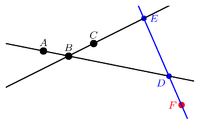Every point F on the line DE belongs to the plane through given points A, B and C, provided that D belongs to the line AB, E belongs to the line BC, and D, E do not coincide with B.

#### Definition via lines

Let three points A, B and C be given, not lying on a line. Consider the lines DE for all points D (different from B) on the line AB and all points E (also different from B) on the line BC. The union of all these lines, together with the point B, is a plane.

This is the plane through A, B and C.

In other words, this plane is the set of all points F such that either F coincides with B or there exists a line through F that intersects the lines AB and BC (in distinct points).

#### Definition via Cartesian coordinates

In terms of Cartesian coordinates x, y, z ascribed to every point of the space, a plane is the set of points whose coordinates satisfy the linear equation

ax + by + cz = d.

Here real numbers a, b, c and d are parameters such that at least one of a, b, c does not vanish.

### Some properties of planes

#### Most basic properties

For any three points not situated in the same straight line there exists one and only one plane that contains these three points.

If (at least) two points of a given straight line lie in a given plane, then all points of this line lie in that plane.

If two planes have a common point then they have (at least) a second point in common.

Every plane contains (at least) three points not lying in the same straight line.

The space contains (at least) four points not lying in the same plane.

#### Further properties

Two planes either do not intersect (are parallel), or intersect in a line, or coincide.

A line either does not intersect a plane (is parallel to it), or intersects it in a single point, or is contained in the plane.

Two lines perpendicular to the same plane are parallel to each other (or coincide).

Two planes perpendicular to the same line are parallel to each other (or coincide).

## Axiomatic approach

### What is wrong with the definitions given above?

The definitions given above assume implicitly that the 3-dimensional Euclidean space is already defined, together with (at least one of) such notions as distances, angles, straight lines, Cartesian coordinates, while planes are not defined yet. However, this situation never appears in mathematical theory.

In the axiomatic approach points, lines and planes are undefined primitives.

The modern approach (below) defines planes in a completely different way.

### How does it work

Axiomatic approach is similar to chess in the following aspect.

A chess piece, say a rook, cannot be defined before the whole chess game is defined, since such a phrase as "the rook moves horizontally or vertically, forward or back, through any number of unoccupied squares" makes no sense unless it is already known that "chess is played on a square board of eight rows and eight columns" etc. And conversely, the whole chess game cannot be defined before each piece is defined; the properties of the rook are an indispensable part of the rules of the game. No chess without rooks, no rooks outside chess! One must introduce the game, its pieces and their properties in a single combined definition.

Likewise, Euclidean space, its points, lines, planes and their properties are introduced simultaneously in a set of 20 assumptions known as Hilbert's axioms of Euclidean geometry. The "most basic properties of planes" listed above are roughly the plane-related assumptions (Hilbert's axioms), while "further properties" are the first plane-related consequences (theorems).

## Modern approach

The modern approach defines the three-dimensional Euclidean space more algebraically, via linear spaces and quadratic forms, namely, as a real affine space whose difference space is a three-dimensional inner product space. For further details see Affine space#Euclidean space and space (mathematics).

In this approach a plane in an n-dimensional affine space (n = 3, or even more) is defined as a two-dimensional affine subspace.

A less formal version of this approach uses points, vectors and scalar product (called also dot product or inner product) of vectors without mentioning linear and affine spaces. Optionally, Cartesian coordinates of points and vectors are used. See algebraic equations below. There, in particular, equivalence between the definition via right angles (orthogonality) and the definition via Cartesian coordinates is explained.

## Plane geometry

Plane geometry (also called "planar geometry") is a part of solid geometry that restricts itself to a single plane ("the plane") treated as a geometric universe. In other words, plane geometry is the theory of the two-dimensional Euclidean space, while solid geometry is the theory of the three-dimensional Euclidean space.

Plane geometry studies the properties of plane figures (and configurations). Plane figures in elementary geometry are sets of points, lines, line segments and sometimes curves that fall on the same plane. For example, triangles, polygons and circles. In plane geometry every figure is plane, in contrast to solid geometry.

## Algebraic equations

In analytic geometry several closely related algebraic equations are known for a plane in three-dimensional Euclidean space. A few algebraic representations will be discussed.

### Point-normal representation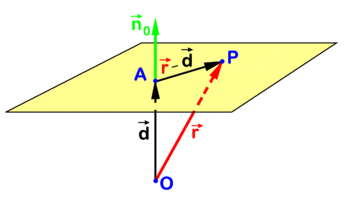Fig. 1. The equation for a plane. P is an arbitrary point in plane. In this picture A is chosen to be closest to the origin O, thus$\scriptstyle\vec{d}$ and$\vec{n}_0$ are collinear.

One such equation is illustrated in Figure 1. Point P runs over the plane; point O is the origin (drawn outside the plane, but can also lie on the plane); A is a given point in the plane (in the picture it is the closest to the origin, but can be chosen arbitrarily); and vector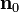$\mathbf{n}_0$ is a unit (length 1) vector normal (perpendicular) to the plane. Taking into account that two nonzero vectors are orthogonal if and only if their scalar product vanishes one gets the following relation (according to the definition via right angles):$(\mathbf{r}-\mathbf{d}) \cdot \mathbf{n}_0 = 0$

where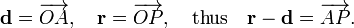$\mathbf{d} = \overrightarrow{OA}, \quad \mathbf{r} = \overrightarrow{OP}, \quad \text{thus} \quad \mathbf{r}-\mathbf{d} = \overrightarrow{AP}.$

(Arrows are dropped from vectors that are now written bold.)

This equation for the plane can be rewritten in terms of coordinates with respect to a Cartesian frame with origin in O: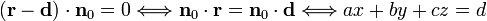$\left( \mathbf{r} - \mathbf{d}\right)\cdot \mathbf{n}_0 = 0 \Longleftrightarrow \mathbf{n}_0 \cdot \mathbf{r} = \mathbf{n}_0 \cdot \mathbf{d} \Longleftrightarrow ax + by + cz = d$

where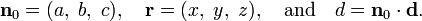$\mathbf{n}_0 = (a,\;b,\; c), \quad \mathbf{r} = (x,\;y,\; z), \quad \text{and} \quad d = \mathbf{n}_0 \cdot \mathbf{d}.$

The definition via Cartesian coordinates is thus derived from the definition via right angles.

The parameters a,b,c,d of the equation do not depend on the choice of the point A on the plane, and satisfy a2 + b2 + c2 = 1. It is convenient to choose A to be the closest to O point of the plane (as shown in Fig. 1). Then the vector$\mathbf{d}$ is perpendicular to the plane and therefore collinear to$\mathbf{n}_0$; together with the relation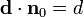$\mathbf{d}\cdot \mathbf{n}_0 = d$ it implies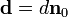$\mathbf{d} = d \mathbf{n}_0$ and so, d is indeed the length of the vector$\mathbf{d}$ as well as the distance of the plane from the origin, provided that$d \ge 0$ (which can be ensured by the right orientation of$\mathbf{n}_0$).

Moreover, the Hesse normal form for the plane (called after the 19th century mathematician Ludwig Otto Hesse) is obtained,$\mathbf{r}\cdot\mathbf{n}_0 = d;$

it is characterized by the use of a unit-length vector$\mathbf{n}_0$ rather than an arbitrary vector orthogonal to the plane.

Conversely, the definition via right angles can be derived from the definition via Cartesian coordinates as follows. Given a linear equation for a plane

ax + by + cz = d

where at least one of a, b, c does not vanish, we may restrict ourselves to the case a2 + b2 + c2 = 1 (since the general case is reduced to this special case via dividing all terms by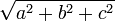$\sqrt{a^2+b^2+c^2}$). Then we introduce$\mathbf{r} = (x,\;y,\; z), \quad\mathbf{n}_0 = (a,\;b,\; c), \quad \mathbf{d} = d \mathbf{n}_0$

(or any other vector$\mathbf{d}$ satistying$\mathbf{d}\cdot \mathbf{n}_0 = d$), and get the same orthogonality relation,$(\mathbf{r}-\mathbf{d})\cdot\mathbf{n}_0 = 0.$

### Three-point representation

Figure 2 shows a plane that by definition passes through three given points A, B, and C that are not on one line. The point P is an arbitrary point in the plane and the reference point O is again drawn outside the plane, but the case that the plane passes through O is not excluded. Referring to the figure we introduce the following definitions$\mathbf{a} = \overrightarrow{OA}, \quad \mathbf{b} = \overrightarrow{OB}, \quad \mathbf{c} = \overrightarrow{OC}, \quad \mathbf{r} = \overrightarrow{OP}.$

A plane, being a 2-dimensional affine space, becomes a 2-dimensional linear space if a chosen point A is treated as its origin. The following two vectors belong to the plane$\mathbf{u} = \overrightarrow{AB}= \mathbf{b}-\mathbf{a} ,\quad \mathbf{v} = \overrightarrow{AC}= \mathbf{c}-\mathbf{a}$

and are non-collinear, therefore linearly independent. It follows that any vector in the plane is some linear combination of these two vectors. (This is also expressed as: Any vector in the plane can be decomposed into components along the two non-collinear vectors). In particular,$\overrightarrow{AP}= \mathbf{r}-\mathbf{a} = \lambda \mathbf{u} + \mu\mathbf{v} \quad \text{for some } \lambda,\mu \in \mathbb{R}.$

The real numbers λ and μ specify the direction (and the length) of$\overrightarrow{AP}$. Hence the following equation for the position vector$\mathbf{r}$ of the arbitrary point P in the plane:$\mathbf{r} = \mathbf{a} + \lambda \mathbf{u} + \mu\mathbf{v}$

is known as the point-direction representation of the plane. This representation is equal to the three-point representation$\mathbf{r} = \mathbf{a}+ \lambda (\mathbf{b}-\mathbf{a}) + \mu(\mathbf{c}-\mathbf{a}),$

where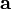$\mathbf{a}$,$\mathbf{b}$, and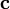$\mathbf{c}$ are the position vectors of the three points that define the plane.

Writing for the position vector of the arbitrary point P in the plane$\mathbf{r} = (1-\lambda-\mu)\, \mathbf{a}+ \lambda\, \mathbf{b} + \mu\,\mathbf{c} \;\equiv\; \xi_1\, \mathbf{a} +\xi_2\,\mathbf{b} + \xi_3\, \mathbf{c}\; ,$

we find that the real triplet (ξ1, ξ2, ξ3) with ξ1 + ξ1 + ξ1 = 1 forms a set of coordinates for P. Such numbers ξ1, ξ2, ξ3 are known as the barycentric coordinates of P. It is trivial to return from barycentric coordinates to the "three-point representation",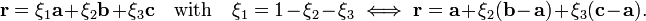$\mathbf{r} = \xi_1 \mathbf{a} + \xi_2\mathbf{b} + \xi_3 \mathbf{c}\quad\hbox{with}\quad \xi_1 = 1- \xi_2-\xi_3 \;\Longleftrightarrow\; \mathbf{r} = \mathbf{a} + \xi_2 (\mathbf{b}-\mathbf{a}) + \xi_3(\mathbf{c}-\mathbf{a}).$

## Beyond mathematics

In industry, a surface plate is a piece of cast iron or other appropriate material whose surface (or rather a part of it) is made as close as possible to a geometric plane (or rather a part of it, usually a square). An old method of their manufacturing is the three-plate method: three roughly flat surfaces become more and more flat when rubbing against each other: first and second; second and third; third and first; first and second again, and so on. It is possible to achieve a surface close to a plane up to 10–5 of its size.

## Reference

1. D. Hilbert, Grundlagen der Geometrie, B. G. Teubner, Leipzig (1899) 2nd German editionSome content on this page may previously have appeared on Citizendium; see the talk page.